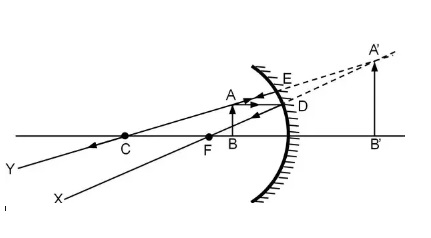# What is the focal length of a makeup mirror that produces a magnification of 1.5 when a person's...

## Question:

What is the focal length of a makeup mirror that produces a magnification of {eq}1.5 {/eq} when a person's face is {eq}12\ \mathrm{cm} {/eq} away? Draw the ray diagram.

## Magnification:

The magnification of the mirror is the property by which the image of the object is enlarged or diminished. If the magnification is more than 1, then the image would be enlarged otherwise it would be diminished.

Given Data:

• Magnification {eq}\rm (m) = 1.5 {/eq}
• Object distance {eq}\rm (u) = 12 \ cm {/eq}

The magnification is given by

{eq}\rm m = \dfrac{-v}{u} \\ 1.5 = \dfrac{-v}{12} \\ v = -18 \ cm {/eq}

Therefore, using the mirror's formula

{eq}\begin{align} \rm \dfrac{1}{f} &= \rm \dfrac{1}{u} + \dfrac{1}{v} \\ \rm \dfrac{1}{f} &= \rm \dfrac{1}{12} + \dfrac{1}{(-18)} \\ \rm f &= \rm 36 \ cm \\ \end{align} {/eq}

Where

• f is the focal length of the mirror